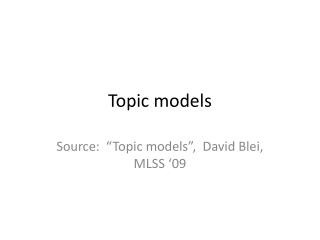# Topic models - PowerPoint PPT PresentationDownload PresentationTopic models

Topic models
Download Presentation## Topic models

- - - - - - - - - - - - - - - - - - - - - - - - - - - E N D - - - - - - - - - - - - - - - - - - - - - - - - - - -
##### Presentation Transcript

1. Topic models Source: “Topic models”, David Blei, MLSS ‘09

2. Topic modeling - Motivation

3. Discover topics from a corpus

4. Model connections between topics

5. Model the evolution of topics over time

6. Image annotation

7. Extensions* • Malleable: Can be quickly extended for data with tags (side information), class label, etc • The (approximate) inference methods can be readily translated in many cases • Most datasets can be converted to ‘bag-of-words’ format using a codebook representation and LDA style models can be readily applied (can work with continuous observations too) *YMMV

8. Connection to ML research

9. Latent Dirichlet Allocation

10. LDA

11. Probabilistic modeling

12. Intuition behind LDA

13. Generative model

14. The posterior distribution

15. Graphical models (Aside)

16. LDA model

17. Dirichlet distribution

18. Dirichlet Examples Darker implies lower magnitude \alpha < 1 leads to sparser topics

19. LDA

20. Inference in LDA

21. Example inference

22. Example inference

23. Topics vs words

24. Explore and browse document collections

25. Why does LDA “work” ?

26. LDA is modular, general, useful

27. LDA is modular, general, useful

28. LDA is modular, general, useful

29. Approximate inference • An excellent reference is “On smoothing and inference for topic models” Asuncion et al. (2009).

30. Posterior distribution for LDA The only parameters we need to estimate are \alpha, \beta

31. Posterior distribution

32. Posterior distribution for LDA • Can integrate out either \theta or z, but not both • Marginalize \theta => z ~ Polya (\alpha) • Polya distribution also known as Dirichlet compound multinomial (models “burstiness”) • Most algorithms marginalize out \theta

33. MAP inference • Integrate out z • Treat \theta as random variable • Can use EM algorithm • Updates very similar to that of PLSA (except for additional regularization terms)

34. Collapsed Gibbs sampling

35. Variational inference Can think of this as extension of EM where we compute expectations w.r.t “variational distribution” instead of true posterior

36. Mean field variational inference

37. MFVI and conditional exponential families

38. MFVI and conditional exponential families

39. Variational inference

40. Variational inference for LDA

41. Variational inference for LDA

42. Variational inference for LDA

43. Collapsed variational inference • MFVI: \theta, z assumed to be independent • \theta can be marginalized out exactly • Variational inference algorithm operating on the “collapsed space” as CGS • Strictly better lower bound than VB • Can think of “soft” CGS where we propagate uncertainty by using probabilities than samples

44. Estimating the topics

45. Inference comparison

46. Comparison of updates MAP VB CVB0 CGS “On smoothing and inference for topic models” Asuncion et al. (2009).

47. Choice of inference algorithm • Depends on vocabulary size (V) , number of words per document (say N_i) • Collapsed algorithms – Not parallelizable • CGS - need to draw multiple samples of topic assignments for multiple occurrences of same word (slow when N_i >> V) • MAP – Fast, but performs poor when N_i << V • CVB0 - Good tradeoff between computational complexity and perplexity

48. Supervised and relational topic models

49. Supervised LDA

50. Supervised LDA